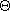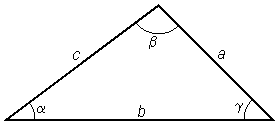## Miscellaneous

 Trigonometry Formulas

### Determining Sides and Angles of a Right Trianglea2 + o2 = h2 (Pythagorean theorem) sin= o csc= 1 = h h sino cos= a sec= 1 = h h cosa tan= o = sincot= 1 = a a costano

### Determining Sides and Angles of Any Trianglesin= sin= sin(Law of Sines) a b c c2 = a2 + b2 - 2ab cos(Law of Cosines)

Known Sides and Angles     Example
All three sides (SSS)
a, b, and c
cos= b2 + c2 - a2

2bc
Two sides and the angle
included between them (SAS)
b,, and c
a2 = b2 + c2 - 2bc cosTwo sides and an angle not
included between them (SSA)
a, b, andsin= b sina
One side and two angles (SAA)
a, andb = a sinsin### Correcting Rotated Graph Axes= tan-1(slope of x) x' = x cos+ y siny' = -x sin+ y cos[  Index  |  Technical Notes Page  ]